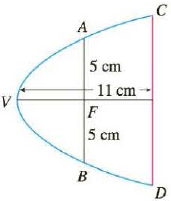Chapter 10.5, Problem 50E

Chapter
Section
Textbook Problem

# A cross-section of a parabolic reflector is shown in the figure. The bulb is located at the focus and the opening at the focus is 10 cm. (a) Find an equation of the parabola. (b) Find the diameter of the opening |CD|, 11 cm from the vertex.a)

To determine

To Find: The equation of the parabola.

Explanation

Given:

The parabola appears as drawn in the below figure.

The opening at the focus is 10cm and distance from the vertex to the CD is 11cm .

Calculation:

Referring to the figure (1), the focus and the vertex are located in the x axis.

The standard equation the parabola is as below.

y2=4px (1)

The focus of the parabola is of the form (p,0) .

Referring to the figure (1) at the point A , the focus of the parabola is (p,5) .

Substitute the value (p,5) for (x,y) in equation (1), to find the value p

b)

To determine

To Find: The diameter of the opening CD.

### Still sussing out bartleby?

Check out a sample textbook solution.

See a sample solution

#### The Solution to Your Study Problems

Bartleby provides explanations to thousands of textbook problems written by our experts, many with advanced degrees!

Get Started

#### Discuss the continuity of f(x)={ x+21x314x2,3x5.

Calculus: An Applied Approach (MindTap Course List)

#### Convert the expressions in Exercises 6584 to power form. 3x25472x3

Finite Mathematics and Applied Calculus (MindTap Course List)

#### In Exercises 110, evaluate the expression. 20!

Finite Mathematics for the Managerial, Life, and Social Sciences

#### The graph of θ = 2 in polar coordinates is a: circle line spiral 3-leaved rose

Study Guide for Stewart's Single Variable Calculus: Early Transcendentals, 8th

#### What is a ceiling effect, and how can it be a problem?

Research Methods for the Behavioral Sciences (MindTap Course List)

#### 66. Solve: 3x2+4=2x.

College Algebra (MindTap Course List)